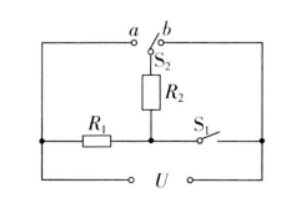(1)请分析论证：当开关S闭合、S2接触点a时，电饭锅处于“超快煮”挡

(2)若电饭锅“标准煮”挡的功率是“保温”挡功率的n倍，求“超快煮”挡功率与“保温”挡功率的比值(1) 当开关 $S_1$ 闭合、 $S_2$ 接触点 $a$ 时, $R_1$ 和 $R_2$ 并联, 总功率 $P_a=\frac{U^2}{R_2}+\frac{U^2}{R_2}$

(2) 根据题意有 $\mathrm{P}_{\mathrm{b}}=n \mathrm{nP}_{\mathrm{b}}{ }^{\prime}$, 即 $\frac{\mathrm{U}^2}{R_1}=n \times=\frac{\mathrm{U}^2}{\mathrm{R}_2+R_2}$, 解得 $R_2=(n-1) R_3$